# JP6393872B2 - Imaging lens - Google Patents

## Info

Publication number
JP6393872B2
JP6393872B2 JP2014172366A JP2014172366A JP6393872B2 JP 6393872 B2 JP6393872 B2 JP 6393872B2 JP 2014172366 A JP2014172366 A JP 2014172366A JP 2014172366 A JP2014172366 A JP 2014172366A JP 6393872 B2 JP6393872 B2 JP 6393872B2
Authority
JP
Japan
Prior art keywords
lens
lt
surface
imaging
imaging lens
Prior art date
Legal status (The legal status is an assumption and is not a legal conclusion. Google has not performed a legal analysis and makes no representation as to the accuracy of the status listed.)
Active
Application number
JP2014172366A
Other languages
Japanese (ja)
Other versions
JP2016048274A5 (en
JP2016048274A (en
Inventor

Original Assignee
カンタツ株式会社
Priority date (The priority date is an assumption and is not a legal conclusion. Google has not performed a legal analysis and makes no representation as to the accuracy of the date listed.)
Filing date
Publication date
Application filed by カンタツ株式会社 filed Critical カンタツ株式会社
Priority to JP2014172366A priority Critical patent/JP6393872B2/en
Publication of JP2016048274A publication Critical patent/JP2016048274A/en
Publication of JP2016048274A5 publication Critical patent/JP2016048274A5/ja
Application granted granted Critical
Publication of JP6393872B2 publication Critical patent/JP6393872B2/en
Active legal-status Critical Current
Anticipated expiration legal-status Critical

• 230000001629 suppression Effects 0.000 description 6
• 230000000694 effects Effects 0.000 description 1
• 239000000203 mixtures Substances 0.000 description 1

## Images

•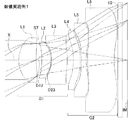•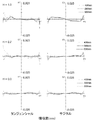•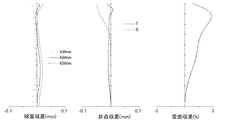••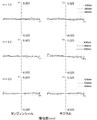••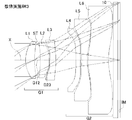••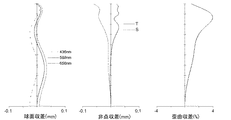•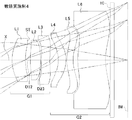•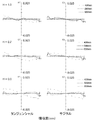•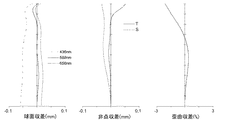•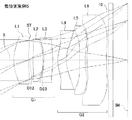•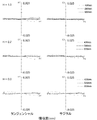•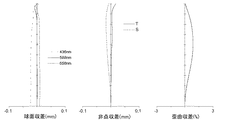•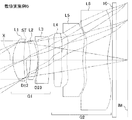•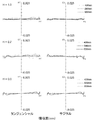•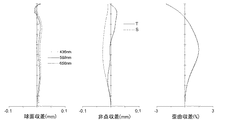## Classifications

• GPHYSICS
• G02OPTICS
• G02BOPTICAL ELEMENTS, SYSTEMS, OR APPARATUS
• G02B13/00Optical objectives specially designed for the purposes specified below
• G02B13/001Miniaturised objectives for electronic devices, e.g. portable telephones, webcams, PDAs, small digital cameras
• G02B13/0015Miniaturised objectives for electronic devices, e.g. portable telephones, webcams, PDAs, small digital cameras characterised by the lens design
• G02B13/002Miniaturised objectives for electronic devices, e.g. portable telephones, webcams, PDAs, small digital cameras characterised by the lens design having at least one aspherical surface
• G02B13/0045Miniaturised objectives for electronic devices, e.g. portable telephones, webcams, PDAs, small digital cameras characterised by the lens design having at least one aspherical surface having five or more lenses
• GPHYSICS
• G02OPTICS
• G02BOPTICAL ELEMENTS, SYSTEMS, OR APPARATUS
• G02B9/00Optical objectives characterised both by the number of the components and their arrangements according to their sign, i.e. + or -
• GPHYSICS
• G02OPTICS
• G02BOPTICAL ELEMENTS, SYSTEMS, OR APPARATUS
• G02B9/00Optical objectives characterised both by the number of the components and their arrangements according to their sign, i.e. + or -
• G02B9/62Optical objectives characterised both by the number of the components and their arrangements according to their sign, i.e. + or - having six components only

## Description

The present invention relates to an imaging lens that forms a subject image on an imaging element such as a CCD sensor or a CMOS sensor, and is a camera, digital still camera, security camera, and in-vehicle camera incorporated in a portable device such as a cellular phone or a portable information terminal. The present invention relates to an imaging lens suitable for incorporation into a relatively small camera such as a network camera.

In recent years, multi-function mobile phones capable of executing various application software in addition to the voice call function, so-called smartphones, have become widespread, instead of mobile phones mainly using voice calls. By executing the application software on the smartphone, functions such as a digital still camera and car navigation can be realized on the smartphone. In order to realize such various functions, smartphones are equipped with cameras in almost all models, similar to mobile phones.

Such smartphone products are often composed of products with various specifications, from beginners to advanced users. Among these, an imaging lens incorporated in a product developed for advanced users is required to have a lens structure having a high resolution that can be used for an imaging element with a higher pixel size as well as a smaller size.

One method for realizing a high-resolution imaging lens is to increase the number of lenses constituting the imaging lens. However, such an increase in the number of lenses tends to increase the size of the imaging lens, which is disadvantageous for incorporation into a small camera such as the smartphone described above. Therefore, conventionally, in the development of imaging lenses, emphasis has been placed on shortening the total track length while increasing the resolution of the imaging lens.

In recent years, with the rapid progress of high-pixel imaging technology and image processing technology, the focus of image pickup lens development is shifting to the realization of high-resolution lens configurations rather than shortening the overall optical length. . For example, in the past, it was common to incorporate a camera unit including an imaging lens and an imaging device inside a smartphone, but recently compared to a digital still camera by attaching a camera unit separate from the smartphone to the smartphone. Attempts have also been made to make it possible to obtain images that are inferior. In other words, by configuring the camera separately from the smartphone, the space limitation when incorporating the camera is solved.

The lens configuration consisting of six lenses is slightly disadvantageous in terms of downsizing the imaging lens because the number of lenses constituting the imaging lens is large. However, since the degree of freedom in design is high, a high-resolution imaging lens is required. It has the potential to achieve a good balance between necessary correction of various aberrations and downsizing of the imaging lens. As such a six-lens imaging lens, for example, an imaging lens described in Patent Document 1 is known.

The imaging lens described in Patent Document 1 includes a positive first lens having a convex surface facing the object side, a negative second lens having a concave surface facing the image surface side, and a negative third lens having a concave surface facing the object side. A lens, a positive fourth lens and a fifth lens having a convex surface facing the image surface side, and a negative sixth lens having a concave surface facing the object side are arranged. In the imaging lens disclosed in Patent Document 1, the conditional expression regarding the ratio between the focal length of the first lens and the focal length of the third lens and the ratio between the focal length of the second lens and the focal length of the entire lens system is satisfied. As a result, good correction of distortion and chromatic aberration is realized.

JP 2013-195587 A

Mobile phones and smartphones are becoming more advanced and smaller in size year by year, and the level of downsizing required for imaging lenses is higher than before. Since the imaging lens described in Patent Document 1 has a long distance from the object-side surface of the first lens to the image plane of the imaging device, it achieves excellent aberration correction while reducing the size of the imaging lens in response to these requirements. There is a limit to this naturally. As described above, there is a method of reducing the level of downsizing of the imaging lens by configuring the camera separately from the mobile phone or smartphone. However, the camera-equipped mobile phone or smartphone is more convenient and portable. Therefore, there is still a strong demand for compact and high-resolution imaging lenses.

These problems are not unique to imaging lenses built into mobile phones and smartphones. Compared to digital still cameras, personal digital assistants, security cameras, in-vehicle cameras, network cameras, etc., which have become increasingly sophisticated and miniaturized in recent years. This is a common problem even in an imaging lens incorporated in a small camera.

An object of the present invention is to provide an imaging lens capable of achieving both a reduction in size of the imaging lens and good aberration correction.

In order to achieve the above object, an imaging lens of the present invention includes a first lens group having a positive refractive power and a second lens group having a negative refractive power in order from the object side to the image plane side. Configured. The first lens group includes a first lens having a positive refractive power, a second lens having a positive refractive power, and a third lens having a negative refractive power. The second lens group includes a fourth lens having a negative refractive power, a fifth lens having a negative refractive power, and a sixth lens having a positive refractive power. In the imaging lens according to the present invention, the focal length of the entire lens system is f, the focal length of the first lens group is F1, the Abbe number of the first lens is νd1, the Abbe number of the second lens is νd2, and the third lens. Where the Abbe number is νd3 , the distance on the optical axis between the first lens and the second lens is D12, and the distance on the optical axis between the second lens and the third lens is D23. Expressions (1) to (4) and (7) are satisfied.
0.6 <F1 / f <1.4 (1)
40 <νd1 <75 (2)
40 <νd2 <75 (3)
15 <νd3 <35 (4)
3 <D12 / D23 <8 (7)

In the imaging lens of the present invention, the first lens group includes three lenses whose refractive power arrangement is positive and negative. These three lenses are formed from lens materials that satisfy the conditional expressions (2) to (4), respectively, and the first and second lenses and the third lens are composed of a low dispersion material and a high dispersion material. It becomes a combination. By such an arrangement of the refractive powers of each lens and the arrangement of Abbe numbers, the occurrence of chromatic aberration is suitably suppressed and the generated chromatic aberration is corrected well in the first lens group. In the imaging lens according to the present invention, since the positive refractive power is shared by the two lenses, the first lens and the second lens, the refractive power of each of the first lens and the second lens is relatively weak. Therefore, it is possible to reduce the size of the imaging lens while favorably correcting various aberrations.

Conditional expression (1) is a condition for suppressing chromatic aberration, astigmatism, and field curvature within a preferable range in a well-balanced manner while reducing the size of the imaging lens. If the upper limit of “1.4” is exceeded, the refractive power of the first lens group becomes relatively weak with respect to the refractive power of the entire lens system, so that the back focal length becomes longer and the imaging lens becomes smaller. It becomes difficult. As for chromatic aberration, although it is advantageous for correction of longitudinal chromatic aberration, lateral chromatic aberration is insufficiently corrected (the image point of short wavelength moves toward the optical axis with respect to the image point of reference wavelength). Further, since the image plane is curved toward the image plane, that is, the field curvature is overcorrected and the astigmatic difference is increased, it is difficult to obtain good imaging performance.

On the other hand, when the value is below the lower limit “0.6”, the refractive power of the first lens group becomes relatively stronger than the refractive power of the entire lens system, which is advantageous for downsizing of the imaging lens. However, the axial chromatic aberration is undercorrected (the focal position of the short wavelength moves to the object side with respect to the focal position of the reference wavelength), and the sagittal image plane of the astigmatism falls to the object side and astigmatism occurs. Therefore, it is difficult to obtain good imaging performance.

Conditional expression (7) is a condition for suppressing field curvature and distortion within a preferable range while reducing the size of the imaging lens. Exceeding the upper limit “8” is advantageous for downsizing the imaging lens, but it is difficult to ensure the back focus. The curvature of field is overcorrected because the tangential image surface of the astigmatism falls to the image surface side. In addition, distortion increases in the positive direction, making it difficult to obtain good imaging performance. On the other hand, when the value is below the lower limit “3”, it is advantageous for securing the back focus, but it is difficult to reduce the size of the imaging lens. In addition, the sagittal image plane of the astigmatism falls to the object side, making it difficult to obtain good imaging performance.

In the imaging lens having the above-described configuration, it is desirable that the following conditional expression (5) is satisfied when the focal length of the first lens is f1 and the focal length of the second lens is f2.
0.5 <f1 / f2 <5.0 (5)

Conditional expression (5) is a condition for favorably correcting chromatic aberration, astigmatism, and field curvature. If the upper limit “5.0” is exceeded, the refractive power of the first lens becomes relatively weaker than the refractive power of the second lens, which is advantageous for securing the back focus, but the imaging lens is downsized. It becomes difficult. Moreover, since the sagittal image plane of the astigmatism is tilted to the image plane side and astigmatism increases, it is difficult to obtain good imaging performance. On the other hand, when the value is below the lower limit “0.5”, the refractive power of the first lens becomes relatively stronger than the refractive power of the second lens, which is advantageous for downsizing the imaging lens. However, the back focus is shortened, and it is difficult to secure a space for placing an insert such as an infrared cut filter. Moreover, since the sagittal image plane of the astigmatism falls to the object side and the astigmatism increases, it is difficult to obtain good imaging performance.

In the imaging lens having the above configuration, it is preferable that the following conditional expression (6) is satisfied when the focal length of the third lens is f3.
−3.5 <f3 / F1 <−0.5 (6)

Conditional expression (6) is a condition for satisfactorily correcting chromatic aberration and astigmatism. If the upper limit “−0.5” is exceeded, the negative refractive power of the third lens in the first lens group becomes relatively strong, so that astigmatism increases toward the image plane side and the magnification of the off-axis light beam. Chromatic aberration is overcorrected (the imaging point with a short wavelength moves in a direction away from the optical axis with respect to the imaging point with a reference wavelength), making it difficult to obtain good imaging performance. On the other hand, if the value is below the lower limit “−3.5”, astigmatism can be easily corrected, but axial chromatic aberration increases, making it difficult to obtain good imaging performance.

In the imaging lens having the above configuration, it is desirable that the following conditional expression (8) is satisfied when the combined focal length of the fourth lens and the fifth lens is f45.
−7.0 <f45 / f <−0.5 (8)

Conditional expression (8) is a condition for satisfactorily correcting chromatic aberration and astigmatism. When the upper limit “−0.5” is exceeded, the negative refractive power composed of the fourth lens and the fifth lens becomes relatively strong with respect to the refractive power of the entire lens system, and therefore the sixth lens in the second lens group. The positive refractive power of becomes relatively strong. For this reason, off-axis lateral chromatic aberration is insufficiently corrected and astigmatism increases, making it difficult to obtain good imaging performance. On the other hand, when the value falls below the lower limit “−7.0”, the negative refractive power composed of the fourth lens and the fifth lens is relatively weak with respect to the refractive power of the entire lens system. However, since the astigmatic difference increases, it is difficult to obtain good imaging performance.

In the imaging lens having the above configuration, it is preferable that the following conditional expression (9) is satisfied when the focal length of the sixth lens is f6.
2 <f6 / f <10 (9)

Conditional expression (9) is a condition for suppressing astigmatism and lateral chromatic aberration within a favorable range. When the upper limit “10” is exceeded, the refractive power of the sixth lens becomes relatively weak with respect to the refractive power of the entire lens system, so that the tangential image surface of the astigmatism is curved to the image surface side and becomes non-symmetrical. The point difference increases, making it difficult to obtain good imaging performance. On the other hand, below the lower limit “2”, the refracting power of the sixth lens becomes relatively strong with respect to the refracting power of the entire lens system, and the lateral chromatic aberration with respect to the off-axis light beam increases. In addition, since the tangential image surface of the astigmatism falls to the object side and the astigmatic difference increases, it is difficult to obtain good imaging performance.

In the imaging lens having the above-described configuration, it is desirable that the following conditional expression (10) is satisfied when the combined focal length of the fourth lens and the fifth lens is f45 and the focal length of the sixth lens is f6.
−0.8 <f45 / f6 <−0.1 (10)

Conditional expression (10) is a condition for correcting distortion, field curvature, and chromatic aberration in a well-balanced manner while reducing the size of the imaging lens. When the upper limit “−0.1” is exceeded, the negative refractive power of the fourth lens and the fifth lens becomes relatively strong with respect to the positive refractive power of the sixth lens. Although advantageous, it is difficult to ensure the back focus. Further, the positive distortion increases and the imaging surface is curved toward the object side, that is, the curvature of field is insufficiently corrected, making it difficult to obtain good imaging performance. On the other hand, when the value falls below the lower limit “−0.8”, the negative refractive power of the fourth lens and the fifth lens becomes relatively weak with respect to the positive refractive power of the sixth lens. Although easy, it is difficult to reduce the size of the imaging lens. Further, the lateral chromatic aberration with respect to the off-axis light beam is insufficiently corrected, and it is difficult to obtain good imaging performance.

In the imaging lens having the above-described configuration, it is desirable that the following conditional expression (11) is satisfied when the curvature radius of the image side surface of the sixth lens is R6r.
0.3 <R6r / f <1.2 (11)

Conditional expression (11) is a condition for suppressing distortion, lateral chromatic aberration, and astigmatism within a preferable range. If the upper limit value “1.2” is exceeded, the lateral chromatic aberration with respect to the off-axis light beam increases, making it difficult to obtain good imaging performance. On the other hand, when the value is below the lower limit “0.3”, the negative distortion increases and the sagittal image surface of the astigmatism falls to the object side and the astigmatism increases. Further, the lateral chromatic aberration with respect to the off-axis light beam is insufficiently corrected, and it is difficult to obtain good imaging performance.

In the present invention, whether the radius of curvature is positive or negative is a general definition, that is, the light traveling direction is positive, and when the center of curvature is on the image plane side when viewed from the lens surface, the radius of curvature is positive. If it is on the side, it follows the definition that the radius of curvature is negative.

In order to further reduce the size of the imaging lens in the imaging lens having the above configuration, it is desirable to satisfy the following conditional expression (12) when the Abbe number of the sixth lens is νd6.
15 <νd6 <35 (12)

According to the imaging lens of the present invention, it is possible to provide a small imaging lens particularly suitable for incorporation into a small camera while having high resolution in which various aberrations are well corrected.

1 is a cross-sectional view illustrating a schematic configuration of an imaging lens according to Numerical Example 1 according to an embodiment of the present invention. FIG. 3 is an aberration diagram illustrating lateral aberration of the imaging lens illustrated in FIG. 1. FIG. 2 is an aberration diagram illustrating spherical aberration, astigmatism, and distortion of the imaging lens illustrated in FIG. 1. It is sectional drawing which shows schematic structure of the imaging lens which concerns on numerical example 2 about one embodiment of this invention. FIG. 5 is an aberration diagram showing lateral aberration of the imaging lens shown in FIG. 4. FIG. 5 is an aberration diagram illustrating spherical aberration, astigmatism, and distortion of the imaging lens illustrated in FIG. 4. It is sectional drawing which shows schematic structure of the imaging lens which concerns on numerical Example 3 about one embodiment of this invention. FIG. 8 is an aberration diagram showing lateral aberration of the imaging lens shown in FIG. 7. FIG. 8 is an aberration diagram illustrating spherical aberration, astigmatism, and distortion of the imaging lens illustrated in FIG. 7. It is sectional drawing which shows schematic structure of the imaging lens which concerns on numerical Example 4 about one embodiment of this invention. FIG. 11 is an aberration diagram illustrating lateral aberration of the imaging lens illustrated in FIG. 10. FIG. 11 is an aberration diagram illustrating spherical aberration, astigmatism, and distortion of the imaging lens illustrated in FIG. 10. FIG. 9 is a cross-sectional view illustrating a schematic configuration of an imaging lens according to Numerical Example 5 according to an embodiment of the present invention. FIG. 14 is an aberration diagram illustrating lateral aberration of the imaging lens illustrated in FIG. 13. FIG. 14 is an aberration diagram illustrating spherical aberration, astigmatism, and distortion of the imaging lens illustrated in FIG. 13. It is sectional drawing which shows schematic structure of the imaging lens which concerns on numerical Example 6 about one embodiment of this invention. FIG. 17 is an aberration diagram illustrating lateral aberration of the imaging lens illustrated in FIG. 16. FIG. 17 is an aberration diagram illustrating spherical aberration, astigmatism, and distortion of the imaging lens illustrated in FIG. 16.

Hereinafter, an embodiment of the present invention will be described in detail with reference to the drawings.

1, 4, 7, 10, 13, and 16 are cross-sectional views illustrating a schematic configuration of an imaging lens according to Numerical Examples 1 to 6 of the present embodiment. Since all the numerical examples have the same basic lens configuration, the lens configuration of the imaging lens according to the present embodiment will be described here with reference to the schematic cross-sectional view of the numerical example 1.

As shown in FIG. 1, the imaging lens according to the present embodiment includes a first lens group G1 having a positive refractive power and a second lens group having a negative refractive power in order from the object side to the image plane side. G2 is arranged and configured. A filter 10 is disposed between the second lens group G2 and the image plane IM of the image sensor. This filter 10 can be omitted.

The first lens group G1 includes, in order from the object side, a first lens L1 having a positive refractive power, an aperture stop ST, a second lens L2 having a positive refractive power, and a third lens having a negative refractive power. L3. In the imaging lens according to the present embodiment, an aperture stop ST is provided on the image side surface of the first lens L1. Note that the position of the aperture stop ST is not limited to the position between the first lens L1 and the second lens L2 as in the imaging lens of Numerical Example 1. For example, the aperture stop ST may be disposed on the object side of the first lens L1. Thus, in the case of a so-called front diaphragm type lens configuration in which the aperture stop ST is disposed on the object side of the imaging lens, the assembling property of the imaging lens can be improved and the manufacturing cost can be reduced. The pre-aperture type lens configuration also has a feature that it is relatively easy to shorten the optical total length of the imaging lens, so it is also an effective lens configuration for incorporation into portable devices such as mobile phones and smartphones that have become widespread in recent years. . On the other hand, a so-called medium-aperture type lens configuration in which an aperture stop ST is disposed between the first lens L1 and the second lens L2 as in Numerical Example 1 is first compared to the optical total length of the imaging lens. Since the effective diameter of the lens L1 is increased, the presence of the imaging lens in the camera is emphasized, and it is possible to appeal to the user about a high-class feeling and high lens performance as part of the design of the camera.

In the first lens group G1, the first lens L1 has a shape in which both the curvature radius r1 of the object side surface and the curvature radius r2 of the image side surface are positive, and has a convex surface on the object side in the vicinity of the optical axis X. It is formed in a shape that becomes a directed meniscus lens. The shape of the first lens L1 is not limited to the shape according to Numerical Example 1. The shape of the first lens L1 may be any shape as long as the curvature radius r1 of the object side surface is positive. Specifically, the shape of the first lens L1 may be a shape in which the radius of curvature r2 is negative and a biconvex lens in the vicinity of the optical axis X.

The second lens L2 has a shape in which both the radius of curvature r3 of the object side surface and the radius of curvature r4 of the image side surface are negative, and is a meniscus lens having a concave surface facing the object side in the vicinity of the optical axis X. It is formed into a shape. The shape of the second lens L2 is not limited to the shape according to the first numerical value example, and may be any shape as long as the radius of curvature r4 of the image side surface is negative. The second lens L2 of Numerical Examples 2, 4, and 5 is an example of a shape having a positive curvature radius r3 and a biconvex lens in the vicinity of the optical axis X.

The third lens L3 is a meniscus lens having a negative curvature radius r5 on the object side surface and a curvature radius r6 on the image side surface, the concave surface facing the object side in the vicinity of the optical axis X. Is formed into a shape. The shape of the third lens L3 is not limited to the shape according to Numerical Example 1. The third lens L3 of Numerical Examples 2 and 6 is an example of a shape in which the radius of curvature r5 is negative and the radius of curvature r6 is positive and becomes a biconcave lens in the vicinity of the optical axis X. The third lens L3 of Example 4 is an example of a shape in which both the radius of curvature r5 and the radius of curvature r6 are positive and a meniscus lens having a convex surface facing the object side in the vicinity of the optical axis X.

The second lens group G2 includes, in order from the object side, a fourth lens L4 having a negative refractive power, a fifth lens L5 having a negative refractive power, and a sixth lens L6 having a positive refractive power. The

In the second lens group G2, the fourth lens L4 has a shape in which both the curvature radius r7 of the object side surface and the curvature radius r8 of the image side surface are negative, and has a concave surface on the object side in the vicinity of the optical axis X. It is formed in a shape that becomes a directed meniscus lens. The shape of the fourth lens L4 is not limited to the shape according to Numerical Example 1. The fourth lens L4 of Numerical Example 3 is an example of a shape in which the radius of curvature r7 is negative and the radius of curvature r8 is positive, and is a biconcave lens in the vicinity of the optical axis X. Numerical Example 5 The fourth lens L4 of Nos. 6 and 6 is an example of a shape in which the curvature radius r7 and the curvature radius r8 are both positive and a meniscus lens having a convex surface facing the object side in the vicinity of the optical axis X.

The fifth lens L5 has a shape in which the curvature radius r9 of the object side surface is negative and the curvature radius r10 of the image side surface is positive, and is a biconcave lens in the vicinity of the optical axis X. . The shape of the fifth lens L5 is not limited to the shape according to Numerical Example 1. The fifth lens L5 of Numerical Examples 2 to 6 has an example in which both the radius of curvature r9 and the radius of curvature r10 are positive, and a meniscus lens having a convex surface facing the object side in the vicinity of the optical axis X. It is.

The sixth lens L6 has a shape in which the curvature radius r11 of the object side surface and the curvature radius r12 of the image side surface are both positive, and is a meniscus lens having a convex surface facing the object side in the vicinity of the optical axis X. It is formed into a shape. In the sixth lens L6, the object side surface and the image side surface are formed in an aspherical shape having inflection points. With such a shape of the sixth lens L6, not only axial chromatic aberration but also off-axis lateral chromatic aberration is corrected well, and the incident angle of the light beam emitted from the imaging lens to the image plane IM is the principal ray angle. It is suitably suppressed within the range of (CRA: Chief Ray Angle).

The imaging lens according to the present embodiment satisfies the following conditional expressions (1) to (11).
0.6 <F1 / f <1.4 (1)
40 <νd1 <75 (2)
40 <νd2 <75 (3)
15 <νd3 <35 (4)
0.5 <f1 / f2 <5.0 (5)
−3.5 <f3 / F1 <−0.5 (6)
3 <D12 / D23 <8 (7)
−7.0 <f45 / f <−0.5 (8)
2 <f6 / f <10 (9)
−0.8 <f45 / f6 <−0.1 (10)
0.3 <R6r / f <1.2 (11)
However,
f: focal length of the entire lens system F1: focal length of the first lens group G1 f1: focal length of the first lens L1 f2: focal length of the second lens L2 f3: focal length of the third lens L3 f6: sixth lens Focal length of L6 f45: Composite focal length of the fourth lens L4 and the fifth lens L5 D12: Distance on the optical axis between the first lens L1 and the second lens L2 D23: Second lens L2 and third lens L3 Νd1: Abbe number of the first lens L1 νd2: Abbe number of the second lens L2 νd3: Abbe number of the third lens L3 R6r: Curvature of the image side surface of the sixth lens L6 radius

In addition, the imaging lenses of Numerical Examples 1 to 5 according to the present embodiment satisfy the following conditional expression (12).
15 <νd6 <35 (12)
However,
νd6: Abbe number of the sixth lens L6

In the imaging lens according to the present embodiment, when emphasizing downsizing of the imaging lens, it is known from the result of optical simulation that satisfying the conditional expression (12) is superior in design. . This is because in the imaging lens according to the present embodiment, since the fourth lens L4 and the fifth lens L5 have negative refractive power, the sixth lens L6 having positive refractive power is formed from a highly dispersed material. However, correction of various aberrations, in particular correction of chromatic aberration, is facilitated.

Note that it is not necessary to satisfy all the conditional expressions, and by satisfying each of the conditional expressions independently, the operational effects corresponding to the conditional expressions can be obtained.

In the present embodiment, the lens surface of each lens is formed as an aspherical surface. The aspherical shape adopted for these lens surfaces is that the axis in the optical axis direction is Z, the height in the direction orthogonal to the optical axis is H, the cone coefficient is k, the aspheric coefficient is A 4 , A 6 , A 8 , When A 10 , A 12 , A 14 , and A 16 are used, they are expressed by the following formula.

Next, numerical examples of the imaging lens according to the present embodiment will be shown. In each numerical example, f represents the focal length of the entire lens system, Fno represents the F number, and ω represents the half angle of view. i is a surface number counted from the object side, r is a radius of curvature, d is a distance (surface interval) between lens surfaces on the optical axis, nd is a refractive index, and νd is an Abbe number. Note that the surface number to which the symbol * (asterisk) is added indicates an aspherical surface.

Numerical example 1
Basic lens data is shown below.
f = 4.89mm, Fno = 2.4, ω = 31.5 °
Unit mm
Surface data Surface number ir d nd νd
(Surface) ∞ ∞
1 * 1.574 0.912 1.5346 56.1 (= νd1)
2 * (Aperture) 10.826 0.295 (= D12)
3 * -4.771 0.473 1.5346 56.1 (= νd2)
4 * -1.685 0.048 (= D23)
5 * -1.795 0.816 1.6355 23.9 (= νd3)
6 * -5.058 0.331
7 * -3.211 0.357 1.5346 56.1 (= νd4)
8 * -4.669 0.054
9 * -12.945 0.256 1.5346 56.1 (= νd5)
10 * 2.882 0.066
11 * 3.249 1.400 1.6142 26.0 (= νd6)
12 * 3.662 (= R6r) 0.200
13 ∞ 0.238 1.5168 64.2
14 ∞ 0.263
(Image plane) ∞

Aspherical data first surface k = 0.000, A 4 = 9.198E-03, A 6 = -6.154E-03, A 8 = -3.882E-03,
A 10 = 2.628E-02, A 12 = -2.072E-02, A 14 = 3.650E-03, A 16 = 2.137E-04
Second side k = 0.000, A 4 = 1.547E-02, A 6 = -1.957E-02, A 8 = 1.607E-02,
A 10 = -3.709E-02, A 12 = 6.649E-03, A 14 = 3.695E-03, A 16 = 1.587E-02
3rd surface k = 0.000, A 4 = -5.861E-02, A 6 = 1.589E-01, A 8 = -5.613E-01,
A 10 = 5.298E-01, A 12 = -5.491E-02, A 14 = -8.742E-02, A 16 = -6.561E-02
4th surface k = 0.000, A 4 = -7.337E-04, A 6 = -9.644E-02, A 8 = 9.046E-02,
A 10 = -6.969E-02, A 12 = 4.672E-02, A 14 = -1.785E-03, A 16 = -5.654E-02
5th surface k = 0.000, A 4 = -1.408E-02, A 6 = -6.729E-02, A 8 = 1.273E-01,
A 10 = -1.282E-01, A 12 = -1.172E-02, A 14 = 1.591E-01, A 16 = -1.749E-01
6th surface k = 0.000, A 4 = 2.366E-03, A 6 = 2.186E-02, A 8 = -3.262E-03,
A 10 = 2.157E-02, A 12 = -7.062E-03, A 14 = -6.923E-03, A 16 = 3.009E-03
7th surface k = 0.000, A 4 = -1.925E-02, A 6 = -7.628E-03, A 8 = 1.018E-02,
A 10 = 7.098E-03, A 12 = -2.338E-03, A 14 = -5.498E-04, A 16 = 7.686E-05
8th surface k = 0.000, A 4 = -4.767E-02, A 6 = 6.429E-03, A 8 = -2.408E-03,
A 10 = 1.420E-03, A 12 = 8.694E-04, A 14 = 1.406E-04, A 16 = -1.457E-04
9th surface k = 0.000, A 4 = -5.522E-02, A 6 = -1.534E-02, A 8 = 2.429E-03,
A 10 = 3.614E-03, A 12 = -1.818E-04, A 14 = -1.730E-04, A 16 = 1.290E-05
10th surface k = 0.000, A 4 = -8.640E-02, A 6 = 5.682E-03, A 8 = 2.436E-03,
A 10 = 4.996E-05, A 12 = -5.492E-05, A 14 = -2.328E-05, A 16 = -2.479E-06
11th surface k = 0.000, A 4 = -9.980E-02, A 6 = 1.842E-02, A 8 = 5.236E-04,
A 10 = -2.064E-04, A 12 = -3.175E-05, A 14 = -9.304E-06, A 16 = -1.065E-06
12th surface k = 0.000, A 4 = -4.166E-02, A 6 = 2.987E-03, A 8 = -4.374E-05,
A 10 = 1.386E-05, A 12 = -1.728E-06, A 14 = -4.773E-07, A 16 = 3.362E-08

f1 = 3.33mm
f2 = 4.63mm
f3 = -4.85mm
f4 = -21.03mm
f5 = -4.38mm
f6 = 20.49mm
f45 = -3.49mm
F1 = 3.71mm

The value of each conditional expression is shown below.
F1 / f = 0.76
f1 / f2 = 0.72
f3 / F1 = -1.31
D12 / D23 = 6.15
f45 / f = -0.71
f6 / f = 4.19
f45 / f6 = -0.17
R6r / f = 0.75
Thus, the imaging lens according to Numerical Example 1 satisfies the above conditional expressions. The distance on the optical axis from the object-side surface of the first lens L1 to the image plane IM (the filter 10 is an air-converted length) is 5.63 mm, and the imaging lens is downsized.

FIG. 2 is an aberration diagram showing the lateral aberration corresponding to the ratio H of each image height to the maximum image height (hereinafter referred to as “image height ratio H”) separately for the tangential direction and the sagittal direction (FIG. 5). The same applies to FIG. 8, FIG. 11, FIG. 14, and FIG. FIG. 3 is an aberration diagram showing spherical aberration (mm), astigmatism (mm), and distortion (%), respectively. In the astigmatism diagrams, S represents a sagittal image plane, and T represents a tangential image plane (the same applies to FIGS. 6, 9, 12, 15 and 18). As shown in FIGS. 2 and 3, the imaging lens according to Numerical Example 1 corrects various aberrations satisfactorily.

Numerical example 2
Basic lens data is shown below.
f = 4.45mm, Fno = 2.4, ω = 34.0 °
Unit mm
Surface data Surface number ir d nd νd
(Surface) ∞ ∞
1 * 1.984 0.813 1.5346 56.1 (= νd1)
2 * (Aperture) 2.544 0.250 (= D12)
3 * 4.699 0.601 1.5346 56.1 (= νd2)
4 * -2.840 0.050 (= D23)
5 * -11.048 0.424 1.6355 23.9 (= νd3)
6 * 5.683 0.453
7 * -1.587 0.390 1.5346 56.1 (= νd4)
8 * -1.781 0.049
9 * 2.374 0.283 1.5346 56.1 (= νd5)
10 * 1.938 0.222
11 * 2.837 1.371 1.6142 26.0 (= νd6)
12 * 2.773 (= R6r) 0.250
13 ∞ 0.200 1.5168 64.2
14 ∞ 0.656
(Image plane) ∞

Aspherical data first surface k = 0.000, A 4 = -4.562E-03, A 6 = -5.283E-03, A 8 = -6.019E-03,
A 10 = 1.512E-02, A 12 = -2.132E-02, A 14 = 1.176E-02, A 16 = -2.562E-03
2nd surface k = 0.000, A 4 = 7.151E-03, A 6 = -2.590E-02, A 8 = -1.896E-03,
A 10 = 7.062E-03, A 12 = -2.034E-02, A 14 = -6.137E-02, A 16 = 7.709E-02
3rd surface k = 0.000, A 4 = -6.912E-03, A 6 = 7.562E-02, A 8 = -3.139E-01,
A 10 = 4.380E-01, A 12 = -2.477E-01, A 14 = -4.975E-02, A 16 = 5.729E-02
4th surface k = 0.000, A 4 = 2.189E-02, A 6 = -1.271E-01, A 8 = 9.746E-02,
A 10 = -7.408E-02, A 12 = 4.872E-02, A 14 = -8.073E-04, A 16 = -2.966E-02
Fifth surface k = 0.000, A 4 = -3.905E-02, A 6 = -1.496E-01, A 8 = 1.412E-01,
A 10 = -8.861E-02, A 12 = -3.623E-02, A 14 = 1.304E-01, A 16 = -8.493E-02
6th surface k = 0.000, A 4 = -5.584E-02, A 6 = -3.533E-03, A 8 = -1.964E-02,
A 10 = 1.995E-02, A 12 = -4.134E-03, A 14 = -5.348E-03, A 16 = 2.536E-03
7th surface k = 0.000, A 4 = 6.106E-02, A 6 = -1.070E-02, A 8 = 1.031E-02,
A 10 = 9.566E-03, A 12 = -5.906E-04, A 14 = -1.078E-04, A 16 = -3.008E-04
8th surface k = 0.000, A 4 = -2.294E-02, A 6 = 9.575E-03, A 8 = 7.558E-03,
A 10 = 4.687E-03, A 12 = 1.223E-03, A 14 = -1.305E-04, A 16 = -3.746E-04
9th surface k = 0.000, A 4 = -6.166E-02, A 6 = -2.713E-02, A 8 = 1.008E-02,
A 10 = -2.386E-04, A 12 = -6.953E-04, A 14 = -6.361E-05, A 16 = 4.752E-05
10th surface k = 0.000, A 4 = -8.046E-02, A 6 = -8.611E-03, A 8 = 4.902E-03,
A 10 = -1.101E-03, A 12 = -5.329E-05, A 14 = -3.142E-05, A 16 = 3.517E-06
11th surface k = 0.000, A 4 = -1.145E-01, A 6 = 3.473E-02, A 8 = -4.742E-03,
A 10 = -4.263E-04, A 12 = -4.328E-05, A 14 = 4.462E-05, A 16 = -5.887E-06
12th surface k = 0.000, A 4 = -6.424E-02, A 6 = 9.466E-03, A 8 = -1.069E-03,
A 10 = -1.307E-05, A 12 = 9.654E-06, A 14 = 2.331E-07, A 16 = -1.228E-07

f1 = 11.19mm
f2 = 3.41mm
f3 = -5.85mm
f4 = -91.28mm
f5 = -25.56mm
f6 = 27.95mm
f45 = -18.52mm
F1 = 4.77mm

The value of each conditional expression is shown below.
F1 / f = 1.07
f1 / f2 = 3.29
f3 / F1 = -1.23
D12 / D23 = 5.00
f45 / f = -4.16
f6 / f = 6.28
f45 / f6 = -0.66
R6r / f = 0.62
Thus, the imaging lens according to Numerical Example 2 satisfies the above conditional expressions. The distance on the optical axis from the object-side surface of the first lens L1 to the image plane IM (the filter 10 is an air-converted length) is 5.94 mm, and the imaging lens is downsized.

FIG. 5 shows lateral aberration corresponding to the image height ratio H for the imaging lens of Numerical Example 2. FIG. 6 shows spherical aberration (mm), astigmatism (mm), and distortion ( %). As shown in FIGS. 5 and 6, various aberrations are favorably corrected by the imaging lens according to Numerical Example 2 as well.

Numerical Example 3
Basic lens data is shown below.
f = 4.13mm, Fno = 2.4, ω = 36.0 °
Unit mm
Surface data Surface number ir d nd νd
(Surface) ∞ ∞
1 * 1.540 0.464 1.5346 56.1 (= νd1)
2 * (Aperture) 4.314 0.296 (= D12)
3 * -8.838 0.470 1.5346 56.1 (= νd2)
4 * -1.831 0.047 (= D23)
5 * -2.295 0.292 1.6355 23.9 (= νd3)
6 * -5.812 1.039
7 * -53.057 0.231 1.5346 56.1 (= νd4)
8 * 13.648 0.061
9 * 85.665 0.296 1.5346 56.1 (= νd5)
10 * 2.489 0.050
11 * 2.627 1.051 1.6142 26.0 (= νd6)
12 * 2.995 (= R6r) 0.250
13 ∞ 0.238 1.5168 64.2
14 ∞ 0.119
(Image plane) ∞

Aspherical data first surface k = 0.000, A 4 = -5.935E-03, A 6 = -9.117E-03, A 8 = -1.112E-02,
A 10 = 1.177E-02, A 12 = -3.881E-02, A 14 = -3.388E-03, A 16 = 1.510E-02
2nd surface k = 0.000, A 4 = -1.025E-02, A 6 = -3.800E-02, A 8 = 8.547E-03,
A 10 = -3.584E-02, A 12 = 1.252E-02, A 14 = 1.376E-02, A 16 = 3.367E-02
3rd surface k = 0.000, A 4 = -8.189E-02, A 6 = 1.798E-01, A 8 = -5.324E-01,
A 10 = 5.535E-01, A 12 = -4.665E-02, A 14 = -8.080E-02, A 16 = -4.768E-02
4th surface k = 0.000, A 4 = -7.818E-03, A 6 = -9.421E-02, A 8 = 9.473E-02,
A 10 = -6.182E-02, A 12 = 5.470E-02, A 14 = -6.955E-03, A 16 = -7.586E-02
Fifth surface k = 0.000, A 4 = -2.114E-02, A 6 = -8.287E-02, A 8 = 1.220E-01,
A 10 = -1.202E-01, A 12 = 6.902E-03, A 14 = 1.668E-01, A 16 = -2.063E-01
6th surface k = 0.000, A 4 = -1.542E-02, A 6 = 2.580E-02, A 8 = -8.636E-04,
A 10 = 1.974E-02, A 12 = -8.650E-03, A 14 = -5.952E-03, A 16 = 7.526E-03
7th surface k = 0.000, A 4 = -5.532E-02, A 6 = -2.350E-02, A 8 = -3.188E-03,
A 10 = 4.416E-03, A 12 = -1.616E-03, A 14 = -2.003E-04, A 16 = 2.989E-04
8th surface k = 0.000, A 4 = -5.211E-02, A 6 = -1.916E-02, A 8 = -2.473E-03,
A 10 = 1.181E-03, A 12 = 8.594E-04, A 14 = 1.698E-04, A 16 = -9.469E-05
9th surface k = 0.000, A 4 = -4.723E-02, A 6 = -1.893E-02, A 8 = 1.185E-03,
A 10 = 3.150E-03, A 12 = 7.818E-05, A 14 = -2.134E-04, A 16 = 1.420E-05
10th surface k = 0.000, A 4 = -8.135E-02, A 6 = 4.227E-03, A 8 = 1.887E-03,
A 10 = -1.201E-05, A 12 = -2.459E-05, A 14 = -1.450E-05, A 16 = 2.312E-07
11th surface k = 0.000, A 4 = -9.707E-02, A 6 = 1.566E-02, A 8 = 2.515E-04,
A 10 = -1.913E-04, A 12 = 4.065E-07, A 14 = -2.711E-06, A 16 = -8.073E-07
12th surface k = 0.000, A 4 = -5.218E-02, A 6 = 4.522E-03, A 8 = 1.842E-05,
A 10 = -4.945E-06, A 12 = -2.208E-06, A 14 = -3.430E-07, A 16 = 2.047E-08

f1 = 4.23mm
f2 = 4.22mm
f3 = -6.17mm
f4 = -20.28mm
f5 = -4.80mm
f6 = 16.68mm
f45 = -3.84mm
F1 = 3.56mm

The value of each conditional expression is shown below.
F1 / f = 0.86
f1 / f2 = 1.00
f3 / F1 = -1.73
D12 / D23 = 6.30
f45 / f = -0.93
f6 / f = 4.04
f45 / f6 = -0.23
R6r / f = 0.72
Thus, the imaging lens according to Numerical Example 3 satisfies the above conditional expressions. The distance on the optical axis from the object-side surface of the first lens L1 to the image plane IM (the filter 10 is an air-converted length) is 4.82 mm, and the imaging lens is downsized.

FIG. 8 shows lateral aberration corresponding to the image height ratio H for the imaging lens of Numerical Example 3, and FIG. 9 shows spherical aberration (mm), astigmatism (mm), and distortion ( %). As shown in FIGS. 8 and 9, various aberrations are also satisfactorily corrected by the imaging lens according to Numerical Example 3 as well.

Numerical Example 4
Basic lens data is shown below.
f = 4.34mm, Fno = 2.4, ω = 34.7 °
Unit mm
Surface data Surface number ir d nd νd
(Surface) ∞ ∞
1 * 2.119 0.693 1.5346 56.1 (= νd1)
2 * (Aperture) 2.922 0.259 (= D12)
3 * 6.115 0.526 1.5346 56.1 (= νd2)
4 * -4.838 0.049 (= D23)
5 * 29.808 0.444 1.6355 23.9 (= νd3)
6 * 7.157 0.439
7 * -1.586 0.394 1.5346 56.1 (= νd4)
8 * -1.776 0.049
9 * 2.273 0.326 1.5346 56.1 (= νd5)
10 * 1.945 0.243
11 * 2.919 1.442 1.6142 26.0 (= νd6)
12 * 2.744 (= R6r) 0.250
13 ∞ 0.200 1.5168 64.2
14 ∞ 0.704
(Image plane) ∞

Aspherical data first surface k = 0.000, A 4 = -4.403E-03, A 6 = -7.214E-03, A 8 = -6.015E-03,
A 10 = 1.512E-02, A 12 = -2.152E-02, A 14 = 1.164E-02, A 16 = -2.353E-03
Second surface k = 0.000, A 4 = 6.162E-03, A 6 = -3.128E-02, A 8 = -4.670E-03,
A 10 = 7.362E-03, A 12 = -1.750E-02, A 14 = -5.933E-02, A 16 = 7.387E-02
Third surface k = 0.000, A 4 = 3.074E-03, A 6 = 6.740E-02, A 8 = -3.162E-01,
A 10 = 4.399E-01, A 12 = -2.449E-01, A 14 = -4.798E-02, A 16 = 5.457E-02
4th surface k = 0.000, A 4 = -1.200E-03, A 6 = -1.288E-01, A 8 = 9.907E-02,
A 10 = -7.458E-02, A 12 = 4.808E-02, A 14 = -1.676E-03, A 16 = -2.982E-02
5th surface k = 0.000, A 4 = -5.300E-02, A 6 = -1.495E-01, A 8 = 1.371E-01,
A 10 = -9.159E-02, A 12 = -3.756E-02, A 14 = 1.309E-01, A 16 = -8.324E-02
6th surface k = 0.000, A 4 = -5.271E-02, A 6 = -1.060E-02, A 8 = -2.073E-02,
A 10 = 2.016E-02, A 12 = -3.924E-03, A 14 = -5.276E-03, A 16 = 2.558E-03
7th surface k = 0.000, A 4 = 5.926E-02, A 6 = -1.017E-02, A 8 = 1.028E-02,
A 10 = 9.421E-03, A 12 = -6.570E-04, A 14 = -1.214E-04, A 16 = -2.892E-04
8th surface k = 0.000, A 4 = -2.381E-02, A 6 = 8.250E-03, A 8 = 7.470E-03,
A 10 = 4.799E-03, A 12 = 1.267E-03, A 14 = -1.239E-04, A 16 = -3.841E-04
9th surface k = 0.000, A 4 = -6.579E-02, A 6 = -2.423E-02, A 8 = 9.859E-03,
A 10 = -3.297E-04, A 12 = -7.230E-04, A 14 = -7.839E-05, A 16 = 3.833E-05
10th surface k = 0.000, A 4 = -8.158E-02, A 6 = -1.080E-02, A 8 = 4.351E-03,
A 10 = -1.257E-03, A 12 = -8.723E-05, A 14 = -3.896E-05, A 16 = 2.306E-06
11th surface k = 0.000, A 4 = -1.138E-01, A 6 = 2.896E-02, A 8 = -5.443E-03,
A 10 = -4.608E-04, A 12 = -3.871E-05, A 14 = 4.778E-05, A 16 = -5.522E-06
12th surface k = 0.000, A 4 = -6.184E-02, A 6 = 8.621E-03, A 8 = -1.011E-03,
A 10 = -7.968E-06, A 12 = 9.849E-06, A 14 = 1.871E-07, A 16 = -1.260E-07

f1 = 11.09mm
f2 = 5.14mm
f3 = -14.93mm
f4 = -99.99mm
f5 = -38.67mm
f6 = 34.86mm
f45 = -25.84mm
F1 = 4.82mm

The value of each conditional expression is shown below.
F1 / f = 1.11
f1 / f2 = 2.16
f3 / F1 = -3.10
D12 / D23 = 5.29
f45 / f = -5.96
f6 / f = 8.04
f45 / f6 = -0.74
R6r / f = 0.63
Thus, the imaging lens according to Numerical Example 4 satisfies the above conditional expressions. The distance on the optical axis from the object side surface of the first lens L1 to the image plane IM (the filter 10 is an air-converted length) is 5.95 mm, and the imaging lens is downsized.

FIG. 11 shows lateral aberration corresponding to the image height ratio H for the imaging lens of Numerical Example 4. FIG. 12 shows spherical aberration (mm), astigmatism (mm), and distortion ( %). As shown in FIGS. 11 and 12, various aberrations are also satisfactorily corrected by the imaging lens according to Numerical Example 4 as well.

Numerical Example 5
Basic lens data is shown below.
f = 5.24mm, Fno = 2.4, ω = 29.8 °
Unit mm
Surface data Surface number ir d nd νd
(Surface) ∞ ∞
1 * 2.172 0.769 1.5346 56.1 (= νd1)
2 * (Aperture) 4.250 0.239 (= D12)
3 * 5.836 0.522 1.5346 56.1 (= νd2)
4 * -3.421 0.064 (= D23)
5 * -2.186 0.459 1.6355 23.9 (= νd3)
6 * -4.857 0.272
7 * 1.936 0.443 1.5346 56.1 (= νd4)
8 * 1.719 0.701
9 * 100.000 0.300 1.5346 56.1 (= νd5)
10 * 3.123 0.097
11 * 4.309 1.017 1.6142 26.0 (= νd6)
12 * 5.968 (= R6r) 0.119
13 ∞ 0.238 1.5168 64.2
14 ∞ 0.768
(Image plane) ∞

Aspherical data first surface k = 0.000, A 4 = -2.088E-02, A 6 = -1.023E-02, A 8 = -8.691E-03,
A 10 = 9.684E-03, A 12 = -1.055E-02, A 14 = 5.032E-03, A 16 = -6.710E-04
Second side k = 0.000, A 4 = -6.691E-02, A 6 = -2.916E-02, A 8 = -8.868E-03,
A 10 = 1.548E-02, A 12 = 3.994E-02, A 14 = -4.925E-02, A 16 = 1.734E-02
3rd surface k = 0.000, A 4 = -7.488E-02, A 6 = -1.912E-02, A 8 = -6.041E-02,
A 10 = 1.502E-01, A 12 = -7.925E-02, A 14 = 1.589E-03, A 16 = 7.000E-03
4th surface k = 0.000, A 4 = -1.569E-02, A 6 = -7.574E-02, A 8 = 1.218E-01,
A 10 = -9.810E-02, A 12 = 9.445E-03, A 14 = 2.847E-02, A 16 = -1.049E-02
5th surface k = 0.000, A 4 = 1.285E-01, A 6 = -1.224E-01, A 8 = 1.366E-01,
A 10 = -1.031E-01, A 12 = -2.725E-02, A 14 = 8.106E-02, A 16 = -2.887E-02
6th surface k = 0.000, A 4 = 5.791E-02, A 6 = -2.348E-02, A 8 = 2.772E-03,
A 10 = 4.828E-03, A 12 = -9.735E-03, A 14 = 1.406E-02, A 16 = -3.998E-03
7th surface k = 0.000, A 4 = -1.189E-01, A 6 = 5.063E-03, A 8 = -1.046E-02,
A 10 = 1.359E-03, A 12 = -9.423E-04, A 14 = 3.641E-03, A 16 = -1.316E-03
8th surface k = 0.000, A 4 = -8.514E-02, A 6 = -9.069E-03, A 8 = 5.478E-04,
A 10 = 1.167E-03, A 12 = 8.254E-05, A 14 = -5.007E-05, A 16 = -4.482E-05
9th surface k = 0.000, A 4 = -5.123E-02, A 6 = 3.968E-03, A 8 = -1.517E-03,
A 10 = -3.192E-04, A 12 = 9.384E-05, A 14 = 3.503E-06, A 16 = 4.323E-05
10th surface k = 0.000, A 4 = -1.110E-01, A 6 = 2.878E-02, A 8 = -8.182E-03,
A 10 = 1.074E-03, A 12 = 5.791E-05, A 14 = 3.028E-05, A 16 = -2.305E-05
11th surface k = 0.000, A 4 = -9.515E-02, A 6 = 1.817E-02, A 8 = -1.045E-03,
A 10 = 1.014E-04, A 12 = -1.021E-04, A 14 = 2.363E-05, A 16 = -8.053E-06
12th surface k = 0.000, A 4 = -4.547E-02, A 6 = 5.453E-03, A 8 = -2.136E-04,
A 10 = -5.780E-05, A 12 = 7.868E-06, A 14 = -6.854E-07, A 16 = 2.237E-08

f1 = 7.36mm
f2 = 4.12mm
f3 = -6.70mm
f4 = -99.75mm
f5 = -6.04mm
f6 = 20.47mm
f45 = -6.10mm
F1 = 4.77mm

The value of each conditional expression is shown below.
F1 / f = 0.91
f1 / f2 = 1.79
f3 / F1 = -1.40
D12 / D23 = 3.73
f45 / f = -1.16
f6 / f = 3.91
f45 / f6 = -0.30
R6r / f = 1.14
Thus, the imaging lens according to Numerical Example 5 satisfies the above conditional expressions. The distance on the optical axis from the object-side surface of the first lens L1 to the image plane IM (the filter 10 is an air-converted length) is 5.93 mm, and the imaging lens is downsized.

FIG. 14 shows lateral aberration corresponding to the image height ratio H for the imaging lens of Numerical Example 5. FIG. 15 shows spherical aberration (mm), astigmatism (mm), and distortion ( %). As shown in FIGS. 14 and 15, various aberrations are also favorably corrected by the imaging lens according to Numerical Example 5.

Numerical Example 6
Basic lens data is shown below.
f = 4.81mm, Fno = 2.4, ω = 32.0 °
Unit mm
Surface data Surface number ir d nd νd
(Surface) ∞ ∞
1 * 1.842 0.575 1.5346 56.1 (= νd1)
2 * (Aperture) 7.266 0.335 (= D12)
3 * -4.093 0.388 1.5346 56.1 (= νd2)
4 * -1.804 0.050 (= D23)
5 * -3.756 0.529 1.6355 23.9 (= νd3)
6 * 14.204 0.250
7 * 8.100 0.412 1.5346 56.1 (= νd4)
8 * 4.557 0.287
9 * 4.075 0.832 1.6142 26.0 (= νd5)
10 * 3.525 0.137
11 * 2.845 1.160 1.5346 56.1 (= νd6)
12 * 2.837 (= R6r) 0.250
13 ∞ 0.200 1.5168 64.2
14 ∞ 0.598
(Image plane) ∞

Aspherical data first surface k = 0.000, A 4 = 2.565E-03, A 6 = -7.583E-03, A 8 = 5.292E-03,
A 10 = 7.267E-03, A 12 = -1.791E-02, A 14 = 8.414E-03, A 16 = -1.337E-03
Second surface k = 0.000, A 4 = -8.223E-03, A 6 = -1.262E-02, A 8 = 7.831E-03,
A 10 = 6.333E-03, A 12 = -9.244E-04, A 14 = -2.581E-02, A 16 = 2.177E-02
3rd surface k = 0.000, A 4 = -5.651E-02, A 6 = 1.536E-01, A 8 = -3.153E-01,
A 10 = 4.294E-01, A 12 = -2.195E-01, A 14 = -2.262E-02, A 16 = 4.223E-02
4th surface k = 0.000, A 4 = 1.180E-01, A 6 = -1.005E-01, A 8 = 1.029E-01,
A 10 = -6.200E-02, A 12 = 4.647E-02, A 14 = -3.884E-02, A 16 = 3.229E-03
Fifth surface k = 0.000, A 4 = 6.450E-02, A 6 = -1.594E-01, A 8 = 1.428E-01,
A 10 = -6.683E-02, A 12 = -7.350E-02, A 14 = 1.102E-01, A 16 = -5.785E-02
6th surface k = 0.000, A 4 = -4.423E-02, A 6 = -2.042E-03, A 8 = -8.354E-03,
A 10 = 1.835E-02, A 12 = -3.205E-03, A 14 = -6.587E-03, A 16 = 2.947E-03
7th surface k = 0.000, A 4 = -5.452E-02, A 6 = -1.950E-04, A 8 = 6.967E-03,
A 10 = 5.352E-03, A 12 = -1.721E-03, A 14 = 3.532E-04, A 16 = -7.046E-05
8th surface k = 0.000, A 4 = -9.743E-02, A 6 = 7.161E-03, A 8 = 2.532E-05,
A 10 = 3.199E-04, A 12 = 4.700E-06, A 14 = -3.275E-05, A 16 = 2.530E-04
9th surface k = 0.000, A 4 = -4.425E-02, A 6 = -2.570E-02, A 8 = 4.511E-03,
A 10 = -3.120E-04, A 12 = -3.774E-03, A 14 = 1.613E-03, A 16 = -4.085E-04
10th surface k = 0.000, A 4 = -6.716E-02, A 6 = 4.534E-03, A 8 = -2.137E-03,
A 10 = -5.641E-06, A 12 = 1.019E-04, A 14 = 2.893E-06, A 16 = 9.273E-07
11th surface k = 0.000, A 4 = -1.273E-01, A 6 = 6.786E-03, A 8 = 2.283E-03,
A 10 = 1.014E-04, A 12 = -7.450E-06, A 14 = -5.377E-06, A 16 = -3.593E-07
12th surface k = 0.000, A 4 = -5.471E-02, A 6 = 1.899E-03, A 8 = 6.800E-04,
A 10 = -1.062E-04, A 12 = 2.142E-06, A 14 = 1.033E-07, A 16 = -9.696E-09

f1 = 4.45mm
f2 = 5.70mm
f3 = -4.62mm
f4 = -20.32mm
f5 = -100.17mm
f6 = 38.10mm
f45 = -15.73mm
F1 = 5.27mm

The value of each conditional expression is shown below.
F1 / f = 1.10
f1 / f2 = 0.78
f3 / F1 = -0.88
D12 / D23 = 6.70
f45 / f = -3.27
f6 / f = 7.93
f45 / f6 = -0.41
R6r / f = 0.59
Thus, the imaging lens according to Numerical Example 6 satisfies the above conditional expressions excluding conditional expression (12). The distance on the optical axis from the object-side surface of the first lens L1 to the image plane IM (the filter 10 is an air-converted length) is 5.93 mm, and the imaging lens is downsized.

FIG. 17 shows lateral aberration corresponding to the image height ratio H for the imaging lens of Numerical Example 6. FIG. 18 shows spherical aberration (mm), astigmatism (mm), and distortion ( %). As shown in FIGS. 17 and 18, various aberrations are favorably corrected also by the imaging lens according to Numerical Example 6.

According to the imaging lens according to the present embodiment described above, a wide angle of view (2ω) of 70 ° or more can be realized. Incidentally, the imaging lenses according to Numerical Examples 1 to 6 described above have a wide angle of view of 59.6 ° to 72.0 °. According to the imaging lens according to the present embodiment, it is possible to capture a wider range than the conventional imaging lens.

In recent years, with the advancement of digital zoom technology for enlarging an arbitrary region of an image obtained through an imaging lens by image processing, a high-pixel imaging element and a high-resolution imaging lens are often combined. In such a high-pixel image sensor, the light-receiving area of each pixel decreases, so that the captured image tends to be dark. As a method for correcting this, there is a method of improving the light receiving sensitivity of the image sensor using an electric circuit. However, when the light receiving sensitivity is increased, noise components that do not directly contribute to image formation are also amplified, so that a new noise reduction circuit is required. Fno of the imaging lenses of Numerical Examples 1 to 6 is as small as 2.4. According to the imaging lens according to the present embodiment, a sufficiently bright image can be obtained without providing the electric circuit or the like.

Therefore, the imaging lens according to the above embodiment is applied to an imaging optical system such as a camera built in a mobile device such as a mobile phone, a portable information terminal, and a smartphone, a digital still camera, a security camera, an in-vehicle camera, and a network camera. In this case, it is possible to achieve both high functionality and downsizing of the camera.

The present invention is applied to an imaging lens incorporated in a relatively small camera such as a camera, a digital still camera, a security camera, an in-vehicle camera, or a network camera incorporated in a portable device such as a mobile phone, a smartphone, or a portable information terminal. Can do.

G1 1st lens group G2 2nd lens group ST Aperture stop L1 1st lens L2 2nd lens L3 3rd lens L4 4th lens L5 5th lens L6 6th lens 10 Filter

## Claims (6)

1. A first lens group having a positive refractive power and a second lens group having a negative refractive power are arranged in order from the object side to the image plane side.
The first lens group includes a first lens having a positive refractive power, a second lens having a positive refractive power, and a third lens having a negative refractive power,
The second lens group includes a fourth lens having a negative refractive power, a fifth lens having a negative refractive power, and a sixth lens having a positive refractive power,
The focal length of the entire lens system is f, the focal length of the first lens group is F1, the Abbe number of the first lens is νd1, the Abbe number of the second lens is νd2, and the Abbe number of the third lens is νd3 . When the distance on the optical axis between the first lens and the second lens is D12, and the distance on the optical axis between the second lens and the third lens is D23 ,
0.6 <F1 / f <1.4
40 <νd1 <75
40 <νd2 <75
15 <νd3 <35
3 <D12 / D23 <8
An imaging lens that satisfies the requirements.
2. When the focal length of the first lens is f1, and the focal length of the second lens is f2,
0.5 <f1 / f2 <5.0
The imaging lens according to claim 1, wherein:
3. When the combined focal length of the fourth lens and the fifth lens is f45,
−7.0 <f45 / f <−0.5
The imaging lens according to claim 1 or 2 , wherein:
4. When the focal length of the sixth lens is f6,
2 <f6 / f <10
The imaging lens according to any one of claims 1 to 3 , wherein:
5. When the combined focal length of the fourth lens and the fifth lens is f45, and the focal length of the sixth lens is f6,
−0.8 <f45 / f6 <−0.1
The imaging lens according to any one of claims 1 to 4, satisfying.
6. When the Abbe number of the sixth lens is νd6,
15 <νd6 <35
The imaging lens according to any one of claims 1 to 5 satisfying.
JP2014172366A 2014-08-27 2014-08-27 Imaging lens Active JP6393872B2 (en)

## Priority Applications (1)

Application Number Priority Date Filing Date Title
JP2014172366A JP6393872B2 (en) 2014-08-27 2014-08-27 Imaging lens

## Applications Claiming Priority (6)

Application Number Priority Date Filing Date Title
JP2014172366A JP6393872B2 (en) 2014-08-27 2014-08-27 Imaging lens
US14/700,211 US9939614B2 (en) 2014-08-27 2015-04-30 Imaging lens for forming an image on an imaging element
CN201520289215.XU CN204613496U (en) 2014-08-27 2015-05-07 Pick-up lens
US15/884,506 US10281689B2 (en) 2014-08-27 2018-01-31 Imaging lens
US15/884,496 US10338349B2 (en) 2014-08-27 2018-01-31 Imaging lens
US15/884,482 US10209493B2 (en) 2014-08-27 2018-01-31 Imaging lens

## Publications (3)

Publication Number Publication Date
JP2016048274A JP2016048274A (en) 2016-04-07
JP2016048274A5 JP2016048274A5 (en) 2017-09-21
JP6393872B2 true JP6393872B2 (en) 2018-09-26

# Family

## Family Applications (1)

Application Number Title Priority Date Filing Date
JP2014172366A Active JP6393872B2 (en) 2014-08-27 2014-08-27 Imaging lens

## Country Status (3)

US (4) US9939614B2 (en)
JP (1) JP6393872B2 (en)
CN (1) CN204613496U (en)

## Families Citing this family (8)

* Cited by examiner, † Cited by third party
Publication number Priority date Publication date Assignee Title
US9772469B2 (en) * 2014-09-17 2017-09-26 Ricoh Company, Ltd. Image forming lens and image capturing device
KR101811570B1 (en) * 2015-11-20 2017-12-22 주식회사 코렌 Photographic lens optical system
KR101883151B1 (en) * 2015-11-26 2018-07-30 삼성전기주식회사 Optical Imaging System
TWI574040B (en) * 2016-04-15 2017-03-11 大立光電股份有限公司 Optical imaging lens assembly, image capturing device and electronic device
TWI616675B (en) * 2016-07-14 2018-03-01 大立光電股份有限公司 Optical photographing assembly, image capturing apparatus and electronic device
TWI637207B (en) 2017-09-22 2018-10-01 大立光電股份有限公司 Imaging lens assembly, image capturing unit and electronic device
CN108761718A (en) * 2018-04-26 2018-11-06 瑞声科技(新加坡)有限公司 Camera optical camera lens
CN108663786A (en) * 2018-04-26 2018-10-16 瑞声科技(新加坡)有限公司 Camera optical camera lens

## Family Cites Families (17)

* Cited by examiner, † Cited by third party
Publication number Priority date Publication date Assignee Title
JP5907417B2 (en) 2012-03-16 2016-04-26 株式会社リコー Imaging lens, imaging device, and information device
JP5755189B2 (en) * 2012-07-02 2015-07-29 キヤノン株式会社 Imaging device and lens device
TWI448725B (en) * 2012-10-22 2014-08-11 Largan Precision Co Ltd Image capturing optical lens system
TWI477803B (en) * 2013-03-05 2015-03-21 Largan Precision Co Ltd Photographing lens assembly
WO2014162779A1 (en) * 2013-04-01 2014-10-09 ソニー株式会社 Image pickup lens and image pickup apparatus
TWI463169B (en) * 2013-07-25 2014-12-01 Largan Precision Co Ltd Image lens assembly and image capturing device
JP2015034922A (en) * 2013-08-09 2015-02-19 日本電産サンキョー株式会社 Wide-angle lens
KR101983147B1 (en) * 2013-08-16 2019-05-28 삼성전기주식회사 Lens module
KR101504035B1 (en) 2013-10-23 2015-03-18 삼성전기주식회사 Lens module
TWI500956B (en) * 2013-11-25 2015-09-21 Largan Precision Co Ltd Photographing lens assembly, image capturing device and mobile terminal
TWI477806B (en) * 2014-01-08 2015-03-21 Largan Precision Co Ltd Photographing optical lens assembly, image capturing device and mobile terminal
TWI480574B (en) * 2014-01-24 2015-04-11 Largan Precision Co Ltd Image capturing lens assembly, image capturing device and mobile terminal
TWI493217B (en) * 2014-05-02 2015-07-21 Largan Precision Co Ltd Photographic optical lens assembly, image capturing unit and mobile device
TWI500961B (en) * 2014-07-04 2015-09-21 Largan Precision Co Ltd Optical imaging lens assembly, image capturing unit and electronic device
TWI500960B (en) * 2014-07-04 2015-09-21 Largan Precision Co Ltd Photographing optical lens system, image capturing unit and mobile terminal
TWI559028B (en) * 2014-07-21 2016-11-21 先進光電科技股份有限公司 Optical image capturing system
KR101649467B1 (en) * 2014-08-11 2016-08-19 주식회사 코렌 Photographic Lens Optical System

## Also Published As

Publication number Publication date
US10209493B2 (en) 2019-02-19
US10281689B2 (en) 2019-05-07
US20160062081A1 (en) 2016-03-03
CN204613496U (en) 2015-09-02
US10338349B2 (en) 2019-07-02
US20180149839A1 (en) 2018-05-31
US20180149841A1 (en) 2018-05-31
US9939614B2 (en) 2018-04-10
US20180149840A1 (en) 2018-05-31
JP2016048274A (en) 2016-04-07

## Similar Documents

Publication Publication Date Title
US9383553B2 (en) Imaging lens and imaging apparatus equipped with the imaging lens
US8749896B2 (en) Imaging lens and imaging apparatus provided with the imaging lens
US8941928B2 (en) Imaging lens and imaging apparatus equipped with the imaging lens
JP6233408B2 (en) Imaging lens and imaging apparatus
US10571663B2 (en) Imaging lens
JP5915462B2 (en) Imaging lens and imaging apparatus
US20190004290A1 (en) Imaging lens
US9304297B2 (en) Imaging lens and imaging apparatus equipped with the imaging lens
US9128267B2 (en) Imaging lens and imaging apparatus including the imaging lens
US9279958B2 (en) Imaging lens and imaging apparatus equipped with the imaging lens
CN106249389B (en) Camera lens
US9423594B2 (en) Imaging lens and imaging apparatus equipped with the imaging lens
JP2016085431A (en) Image capturing lens
JP5435072B2 (en) Imaging lens, imaging device, and portable terminal
JP6105317B2 (en) Wide-angle imaging lens
US9664880B2 (en) Imaging lens and imaging apparatus including the imaging lens
JP5698872B2 (en) Imaging lens and imaging device provided with imaging lens
JP2015141267A (en) Imaging lens and imaging device including the imaging lens
JP5754670B2 (en) imaging lens
JP5750698B2 (en) imaging lens
JP2016095460A (en) Imaging lens and imaging apparatus including imaging lens
JP5904623B2 (en) Imaging lens and imaging device provided with imaging lens
JP5607398B2 (en) Imaging lens, imaging device, and portable terminal device
JP5201690B2 (en) Imaging lens
US9235200B2 (en) Imaging lens and imaging apparatus equipped with the imaging lens

## Legal Events

Date Code Title Description
A521 Written amendment

Free format text: JAPANESE INTERMEDIATE CODE: A523

Effective date: 20170804

A621 Written request for application examination

Free format text: JAPANESE INTERMEDIATE CODE: A621

Effective date: 20170807

A521 Written amendment

Free format text: JAPANESE INTERMEDIATE CODE: A821

Effective date: 20170807

A977 Report on retrieval

Free format text: JAPANESE INTERMEDIATE CODE: A971007

Effective date: 20180413

TRDD Decision of grant or rejection written
A01 Written decision to grant a patent or to grant a registration (utility model)

Free format text: JAPANESE INTERMEDIATE CODE: A01

Effective date: 20180423

A711 Notification of change in applicant

Free format text: JAPANESE INTERMEDIATE CODE: A712

Effective date: 20180522

A61 First payment of annual fees (during grant procedure)

Free format text: JAPANESE INTERMEDIATE CODE: A61

Effective date: 20180522

A711 Notification of change in applicant

Free format text: JAPANESE INTERMEDIATE CODE: A711

Effective date: 20180704

R150 Certificate of patent or registration of utility model

Ref document number: 6393872

Country of ref document: JP

Free format text: JAPANESE INTERMEDIATE CODE: R150

S531 Written request for registration of change of domicile

Free format text: JAPANESE INTERMEDIATE CODE: R313531

R350 Written notification of registration of transfer

Free format text: JAPANESE INTERMEDIATE CODE: R350